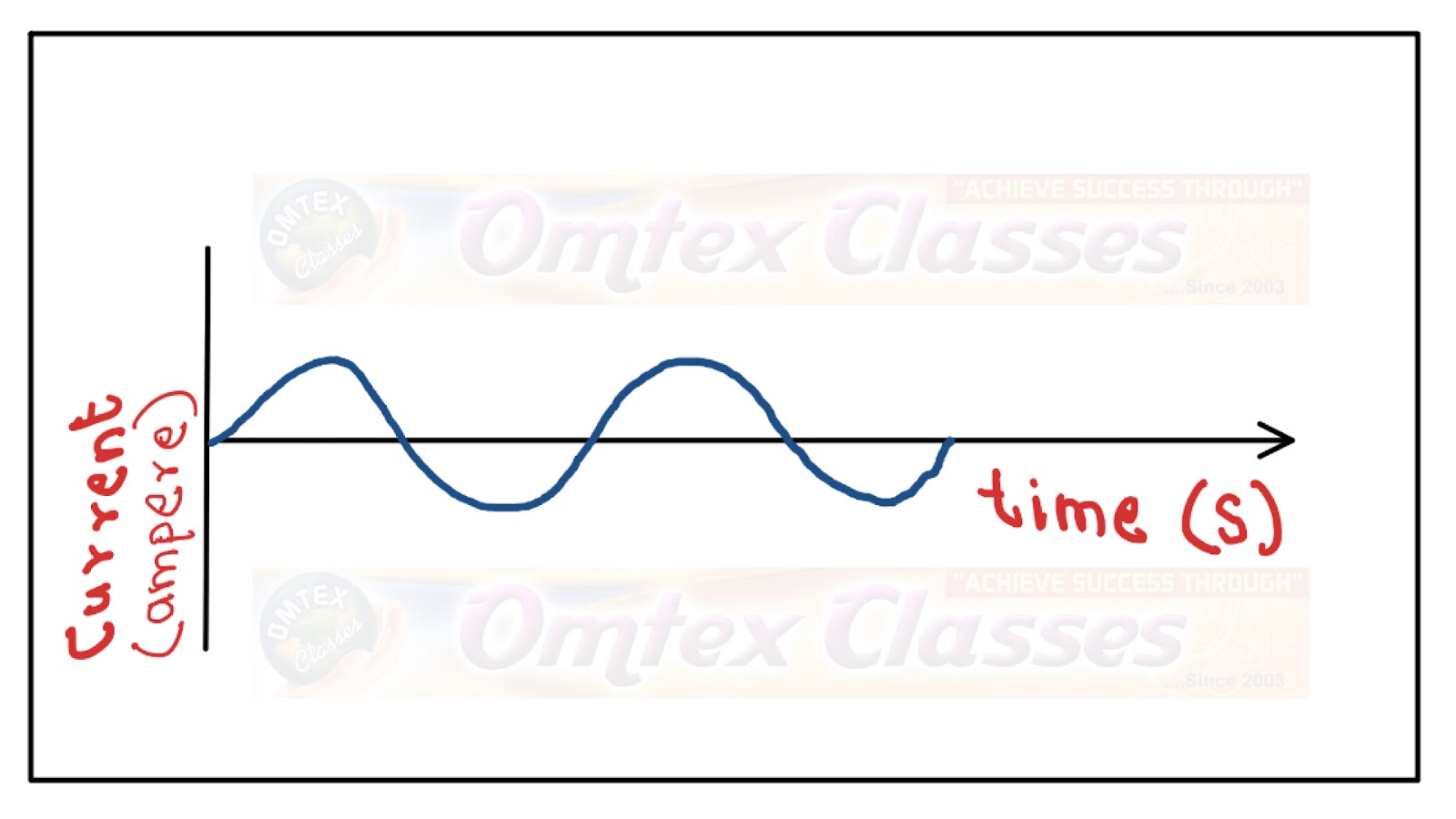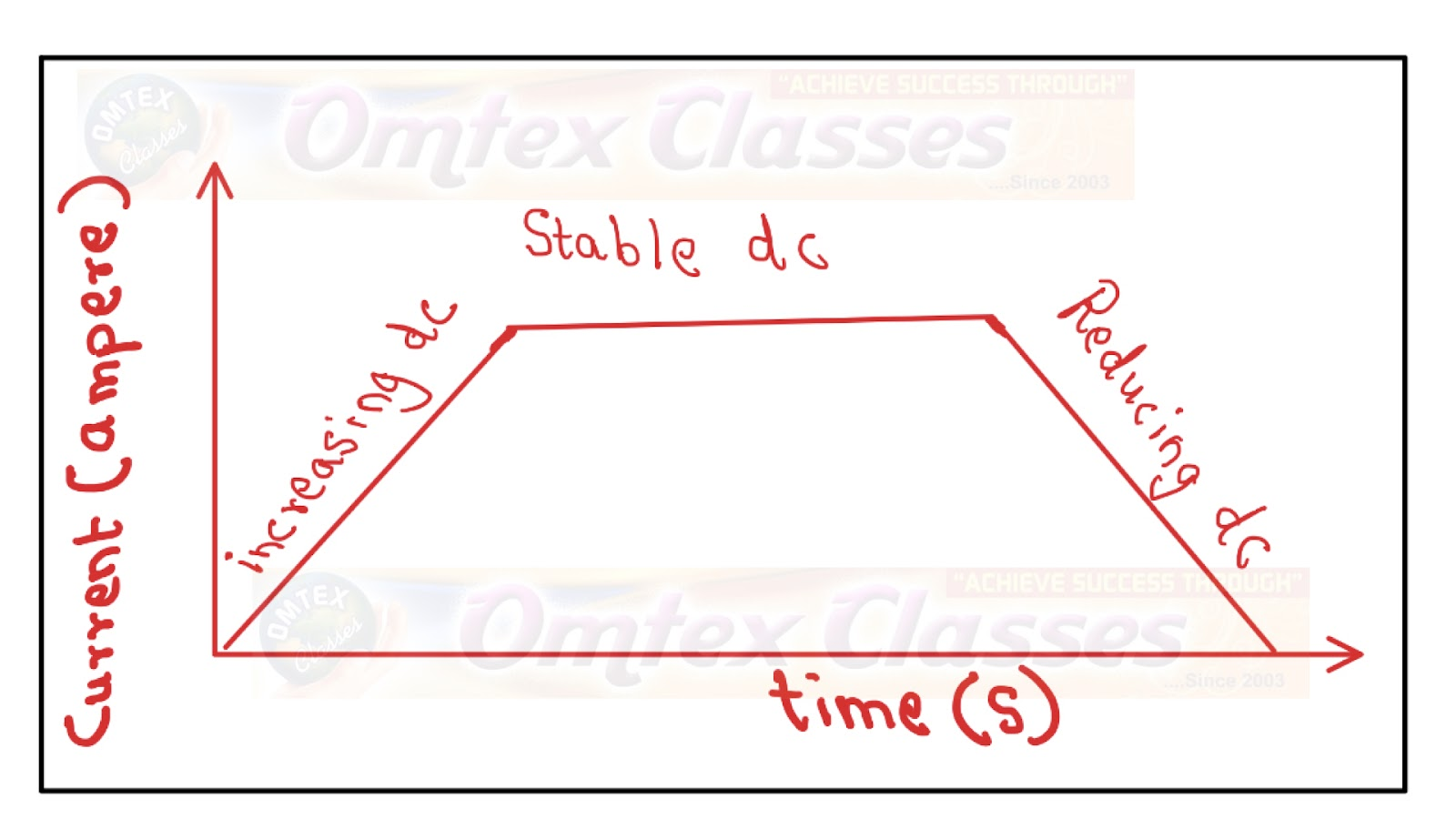9TH MAHARASHTRA: MATHS I 10TH TAMIL NADU: MATHS, SCIENCE, SSLC 10TH NEW TEXT BOOK PDF DOWNLOAD CBSE 10TH: MATHS 2019 BOARD PAPER SOLUTION: 2019 BOARD PAPER SOLUTION

What are the difference between alternating current and direct current?

Chapter No. 4. Effect of Electric Current,

What are the difference between alternating current and direct current?

Ans.

 Alternating current Direct Current Alternating current varies in magnitude continuously, i.e., oscillatory. Magnitude of direct current from a source remains same, i.e., non oscillatory. Direction reverses periodically, i.e. after equal intervals of time. Direction remains same. This type of current is used in electrical household appliances such as electric heater, refrigerator, electric iron, etc. This type of current is not used much for household purposes but used in batteries, torches, etc. Frequency of A.C. in India is 50 Hz, i.e., its cycle changes in 1/50 second. Frequency of D.C. is zero. Produced by A.C. generator. Produced by D.C. generator. Graphical Representation:Graphical representation: